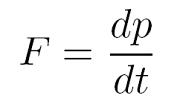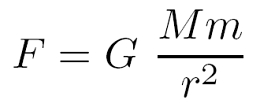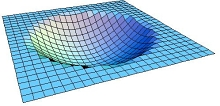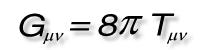# How the UNIVERSAL GRAVITATIONAL constant is actually found

## Gravity

(taken from The classics of physics, P. 334, Verlag Hoffmann and Campe, 2004)

### Newtonian gravity

The study of gravity has been carried out by the English polymath Sir Isaac Newton (1643 - 1727) achieved a major breakthrough. Newton became famous for many achievements: the foundation of differential and integral calculus, discoveries in optics (color theory, corpuscular theory of light) and the theory of gravity that is named after him today. TheNewtonian gravitational physics is the first theory of gravity to deserve the name theory because it is a consistent, comprehensive concept and not just phenomenology or hypothesis. Newton introduced this theory in his work Philosophiae naturalis principia mathematica this work Principia is the very first standard work in theoretical physics! The title is a replica of that Principia Philosophiae of René Descartes.Newton devoted himself to optics and gravity in 1665. The story of the tree falling apple, who hit Newton in the head and inspired him to gravity theory is arguably a myth - Newton only wrote that a falling apple made him think about gravity. At the age of 27, Newton became (on the recommendation of the predecessor of this office) Lukas professor of mathematics - a chair at Cambridge University, which incidentally today Stephen Hawking holds. Newton knew the astronomical observations from Johannes Kepler and his discovery that the planets move on elliptical orbits around the sun. In 1666 Newton began to look for a physical explanation of these purely empirical Kepler laws. Kepler's second law (Area set) he was able to explain in 1679 that there was a attractive central force must give that emanates from the sun.
A meeting of three members of the Royal Society The key event in 1684 was to be: This is where Newton's adversaries metRobert Hooke, the astronomer Edmond Halley and the architect Christopher Wren. They discussed a force that is proportional to the inverse square of the distance and that determines the planetary motion. Inspired by this discussion, Halley asked Newton about the shape of the orbit of a celestial body that results from this law of force. Newton had already calculated this question years earlier and knew that it had to be an elliptical orbit. The detailed elaboration of this calculation ultimately led to Newton's one and a half year creative phase and the publication of the Principia 1687.The book I the Principia contains the three laws of motion nowadays known as Newton's Laws be taught, namely thatLaw of inertia, the dynamic constitution and the Reaction principle (actio = reactio). The dynamic basic law shown in the equation on the left is much better known in school physics F = m a, but this case only applies if the mass is timeU.Ndependent, mm (t), is. In general, however, this is not the case (e.g. with the so-calledRocket equation: a flying missile loses fuel and therefore mass), so the law as the time derivative of the momentum p must be formulated. Book II is a textbook on fluid mechanics. Finally, Newton introduces his be in Book IIILaw of gravity and demonstrates the validity of this theory of gravity using the movements of planets and comets.Newton's theory also states that Instantaneous gravity spread, i.e. without delay. Besides, time and space have one absolute character in Newtonian physics. In the next section we will see that these properties of gravity turn out to be untenable and that Newtonian gravity must be generalized to Einstein's gravity.

### Einstein's general theory of relativity

Presented in 1916 Albert Einstein (1879 - 1955) a completely new view of gravity. In that year he published the General Theory of Relativity (ART), an (unquantized) theory of gravity that describes gravity not as a force but as ageometric property of space and time understand. This theory of gravity was preceded by the special theory of relativity (SRT), which is not a theory of gravity, but which introduced the revolutionary redefinition of the terms energy, mass, time and space.According to Einstein, space and time are linked to one another to form a four-dimensional structure: space-time. While spacetime is still flat in the SRT, it is curved in the GTR. How to simplify in a two-dimensional variant a curved spacetime can imagine, shows the figure on the left. The 'bump' in this 2D space-time is caused by masses. Einstein already recognized in the SRT that energy and mass are equivalent, which in the famous formula E = mc2 is summarized. The consequence: masses and all forms of energy cause 'bumps' in spacetime. A (force-free) movement through space-time is now not possible in any form, but only along certain curves, which in the GTRGeodesics to be named. The geodesics 'meander' through the 'dented spacetime' - exactly how this happens is dictated by the mathematical laws of differential geometry. We take the movement along the geodesics as gravitation. So we move daily under the influence of the curved space-time!The achievement of abstraction is enormous in order to be able to halfway grasp this new picture of gravity. Nobody can imagine a four-dimensional, curved spacetime, but if one suppresses some dimensions, at least one can Space-time diagrams draw that will help you understand. Einstein's new theory of gravity can be summed up in a single but unfortunately complicated formula: the Field equations of the ART. This clear equation is on the right (here for the sake of simplicity without the cosmological constant Λ). In this concise form, the formula directly states that the curved spacetime that is in G is, of mass and energy that is in T stuck, is caused; the equation also includes the aspect that the bumps in spacetime dictate motion. However, the simplicity of this formula is deceptive! Behind the symbols G and T hide math objects that Tensors to be named. More precisely is G the Einstein tensor and T is called the energy-momentum tensor. In general, these objects are very complicated, consist of several components and vary from spacetime point to spacetime point.
A gravitational field in Newtonian physics is now replaced by a space-time in Einsteinian physics. Spacetime is the solution of Einstein's field equation and is clearly described by the metric (the metric tensor G) or alternatively by the line element. The gravitational force of Newton is replaced by the Christoffel symbols, the Riemann tensor and other mathematical quantities of ART (1-forms, 2-forms, Killing fields, Kretschmann scalar, Ricci tensor, Ricci scalar etc.). It is often of interest how test objects move through a curved space-time. To solve this problem, the Geodesic equation be solved. For light, this process is called relativistic ray tracing.
Spacetime are in general too dynamic, i.e. they are constantly changing their curvature properties. This happens especially with Gravitational waveswhich can also be described with Einstein's theory. They are bumps in space-time that move with the speed of light in a vacuum c spread. This is an important difference to Newtonian physics: In Einstein's theory, gravitation does not propagate arbitrarily quickly, but precisely with it c out.
Einstein's ART has proven itself many times in experiments. A number of experimental tests and successes as well as the theory itself are presented in great detail in the dictionary entry General Theory of Relativity.

### relativistic astrophysics

Einstein's theory also had a strong influence on astronomy: Many astrophysical processes can only be treated satisfactorily with Einstein's gravity. The gravitational collapse into a compact object (e.g. neutron stars) is an example of this. Models based on the ART have been established in accretion physics. But even on the very large spatial scale, Einstein's theory is the right choice: cosmology is relativistic. The entire universe can also be understood as a solution to Einstein's field equation and described using the Robertson-Walker metric. The Friedmann world models that emerge from the GTR describe an excellent number of observations of experimental cosmology (see also cosmic background radiation, supernovae type Ia). A number of observed phenomena cannot be understood in the context of Newtonian gravity, e.g. gravitational and cosmological time dilation, redshift and gravitational redshift as well as frame dragging.

### cosmological significance of gravity

The importance of gravity for the entire universe is based on two properties that were already mentioned at the beginning: In principle, gravity has one any range, And she is not shield. Due to the first property, gravity can also act over extremely large distances - up to billions of light years, i.e. scales as large as the cosmos itself. Due to the second property, which cannot be shielded, there is hardly any possibility of stopping gravitation (unless through Antigravity - more on this in the next section). The consequence is what astrophysicists do gravitational instability call. One can clearly describe this in such a way that the matter begins to 'clump' due to the influence of gravity: If the jeans mass is exceeded locally for a mass accumulation, gravitational collapse begins. Later this lump fragments into smaller ones. These scenarios are essential to the origin of the spacious structure to understand in the cosmos. From the initial distribution of the 'primordial gas', which arose in the primordial nucleosynthesis, the first generation of stars (Population III) formed through gravitational instabilities. Due to the influence of gravity, the first galaxies arose from this - at least that's how it went in one of them hierarchical growth scenario from.

### Antigravity

Relativistic cosmology has spawned a form of gravity that makes science fiction fans' eyes wet: Antigravity. To explain the context, we need to take a look at modern cosmology. The dynamics of the Friedmann world models already mentioned are determined by the forms of energy in the cosmos. The 'normal', baryonic matter, of which we are made up, is a form of energy, but its meaning is practically irrelevant. Another form of matter, dark matter, is much more common in the universe. It influences not only the dynamics and development of galaxies, but also of the cosmos as a whole. Their relative share is around a third. What exactly is hidden behind dark matter, however, is a mystery - the current ideas are presented in the entry Dark Matter.
Now we come to the crucial part: the remaining two thirds of the energy in the universe is accounted for by the Dark energy. What exactly is behind this mysterious, cosmic ingredient is an explosive and highly topical research area. It is not a concrete form of matter, but rather the physicists favor that the quantum vacuum, which is finely distributed throughout the universe, manifests itself as a cosmologically relevant form of energy. The astronomical observation fact is one accelerated expansion of the cosmos. In the standard model of cosmology this is explained by the existence of dark energy (alternatives are shown in the entry dark energy).
The special thing about dark energy is its equation of state because it unites negative pressure having. That sounds very unphysical, but it explains the observations surprisingly well. According to astronomical observations, the so-called w-parameter is close to w = -1. Thus, from a whole range of models for dark energy, a certain one is obviously preferred by nature, namely thecosmological constantwhich none other than Einstein introduced in 1917. It just has the right w-parameter - and it does not vary over time, which also fits with current observations on distant, exploding white dwarfs. Such an equation of state ensures an expansion of the universe, which can be understood as antigravity. Because dark energy counteracts gravity. Using the Friedmann equation one can show that towards later development epochs the influence of the anti-gravitational dark energy increases. The current data predicts an eternal and ever faster expansion of the universe. In the end there is a cold cosmos, in which probably only black holes (with different masses and angular momentum) and maybe black dwarfs still exist.